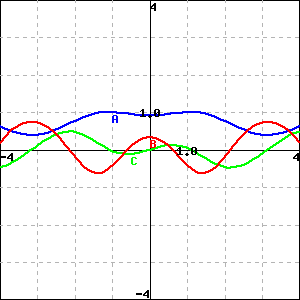Identify the graphs A (blue), B(red) and C (green) as the graphs of a function $f(x)$ and its derivatives $f'(x)$ and $f''(x)$.
(Clicking on the sketch will give you a version of the picture in a separate window.)

is the graph of the function, $f(x)$ .
is the graph of the function's first derivative, $f'(x)$ .
is the graph of the function's second derivative, $f''(x)$ .

Hint: Remember that $f'(x)$ is itself a function, and we can find the derivative of the function $f'(x)$, which is called the second derivative of the function $f(x)$ and denoted by $f''(x)$.

You can earn partial credit on this problem.